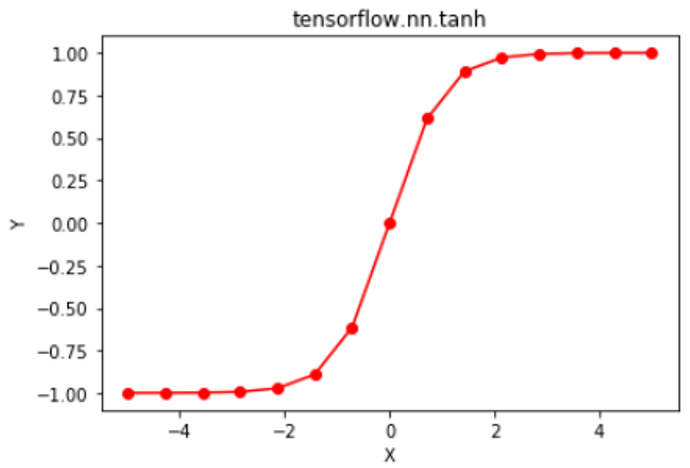# Python | Tensorflow nn.tanh()

Tensorflow is an open-source machine learning library developed by Google. One of its applications is to develop deep neural networks.

The module `tensorflow.nn` provides support for many basic neural network operations.

One of the many activation functions is the hyperbolic tangent function (also known as tanh) which is defined as.

The hyperbolic tangent function outputs in the range (-1, 1), thus mapping strongly negative inputs to negative values. Unlike the sigmoid function, only near-zero values are mapped to near-zero outputs, and this solves the “vanishing gradients” problem to some extent. The hyperbolic tangent function is differentiable at every point and its derivative comes out to be. Since the expression involves the tanh function, its value can be reused to make the backward propagation faster.

Despite the lower chances of the network getting “stuck” when compared with the sigmoid function, the hyperbolic tangent function still suffers from “vanishing gradients”. Rectified Linear Unit (ReLU) can be used to overcome this problem.

The function `tf.nn.tanh()` [alias `tf.tanh`] provides support for the hyperbolic tangent function in Tensorflow.

Syntax: tf.nn.tanh(x, name=None) or tf.tanh(x, name=None)

Parameters:
x: A tensor of any of the following types: float16, float32, double, complex64, or complex128.
name (optional): The name for the operation.

Return : A tensor with the same type as that of x.

Code #1:

 `# Importing the Tensorflow library ` `import` `tensorflow as tf ` ` `  `# A constant vector of size 6 ` `a ``=` `tf.constant([``1.0``, ``-``0.5``, ``3.4``, ``-``2.1``, ``0.0``, ``-``6.5``], dtype ``=` `tf.float32) ` ` `  `# Applying the tanh function and ` `# storing the result in 'b' ` `b ``=` `tf.nn.tanh(a, name ``=``'tanh'``) ` ` `  `# Initiating a Tensorflow session ` `with tf.Session() as sess: ` `    ``print``(``'Input type:'``, a) ` `    ``print``(``'Input:'``, sess.run(a)) ` `    ``print``(``'Return type:'``, b) ` `    ``print``(``'Output:'``, sess.run(b)) `

Output:

```Input type: Tensor("Const_2:0", shape=(6, ), dtype=float32)
Input: [ 1.        -0.5        3.4000001 -2.0999999  0.        -6.5      ]
Return type: Tensor("tanh_2:0", shape=(6, ), dtype=float32)
Output: [ 0.76159418 -0.46211717  0.9977749  -0.97045201  0.         -0.99999547]
```

Code #2: Visualization

 `# Importing the Tensorflow library ` `import` `tensorflow as tf ` ` `  `# Importing the NumPy library ` `import` `numpy as np ` ` `  `# Importing the matplotlib.pylot function ` `import` `matplotlib.pyplot as plt ` ` `  `# A vector of size 15 with values from -5 to 5 ` `a ``=` `np.linspace(``-``5``, ``5``, ``15``) ` ` `  `# Applying the tanh function and ` `# storing the result in 'b' ` `b ``=` `tf.nn.tanh(a, name ``=``'tanh'``) ` ` `  `# Initiating a Tensorflow session ` `with tf.Session() as sess: ` `    ``print``(``'Input:'``, a) ` `    ``print``(``'Output:'``, sess.run(b)) ` `    ``plt.plot(a, sess.run(b), color ``=` `'red'``, marker ``=` `"o"``)  ` `    ``plt.title(``"tensorflow.nn.tanh"``)  ` `    ``plt.xlabel(``"X"``)  ` `    ``plt.ylabel(``"Y"``)  ` ` `  `    ``plt.show() `

Output:

```Input: [-5.         -4.28571429 -3.57142857 -2.85714286 -2.14285714 -1.42857143
-0.71428571  0.          0.71428571  1.42857143  2.14285714  2.85714286
3.57142857  4.28571429  5.        ]
Output: [-0.9999092  -0.99962119 -0.99842027 -0.99342468 -0.97284617 -0.89137347
-0.61335726  0.          0.61335726  0.89137347  0.97284617  0.99342468
0.99842027  0.99962119  0.9999092 ]
```My Personal Notes arrow_drop_upCheck out this Author's contributed articles.

If you like GeeksforGeeks and would like to contribute, you can also write an article using contribute.geeksforgeeks.org or mail your article to contribute@geeksforgeeks.org. See your article appearing on the GeeksforGeeks main page and help other Geeks.

Please Improve this article if you find anything incorrect by clicking on the "Improve Article" button below.

Improved By : sanskar27jain

Article Tags :

Be the First to upvote.

Please write to us at contribute@geeksforgeeks.org to report any issue with the above content.